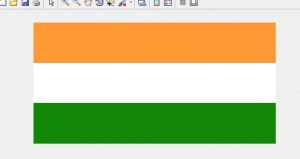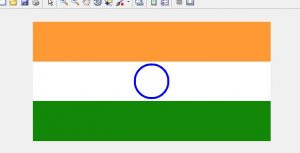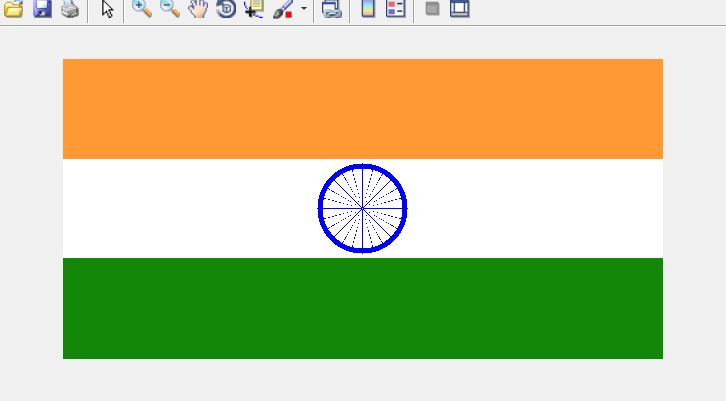# Draw Indian Flag using matlab

In Digital image processing, a colored image is represented in a 3-Dimensional matrix. Image can be represented in various color models such as RGB (Red, Green, Blue) model, HSV (Hue, Saturation, Value) model, YIQ (Luminance-Inphase Quadrature) model, CMYK (Cyan, Magenta, Yellow, Black) model. Generally, an image is represented in the RGB model. The first channel of the matrix is Red, the second channel is the Green and the third channel is Blue.

Approach to draw Indian Flag

• Firstly, we need to create a matrix of 300X600X3 and fill the matrix with white color. 300 is the number of rows and 600 is the number of columns. For color image 3 channels are required, so 3 represents RGB(Red Green Blue) channel.
`img=uint8(zeros(300, 600, 3))`
• Divide the matrix into three parts (0-100)rows for saffron color, (101-200)rows for white color and ashok chakra, (201-300)rows for green.
• Fill the matrix with saffron color(255, 153, 51) from 1st row to 100th row.
```img(1:100, 1:600, 1)=255;
img(1:100, 1:600, 2)=153;
img(1:100;1:600, 3)=51;
```
• Fill the matrix with green color(19, 136, 8) from row 201 to 300.
```img(201:300, 1:600, 1)=19;
img(201:300, 1:600, 2)=136;
img(201:300, 1:600, 3)=8;
```
•• To make ashok chakra, first we need to understand the equation of distance between two given coordinates.
`(distance)2=(x2-x1)2+(y2-y1)2`

(x1, y1) and (x2, y2) are the two given coordinates.

• Using the above equation, we can make the circle of ashok chakra. Center coordinates of the matrix and circle is (150, 300). To draw cirle inner radius is 40 and outer radius is 45.
•  `for` `i``=``1``:``300` `       ``for` `j``=``1``:``600` `           ``if` `(sqrt(power(``150``-``i, ``2``)``+``power(``150``-``j, ``2``))>``=``40` `&& ` `               ``sqrt(power(``150``-``i,``2``)``+``power(``150``-``j,``2``))<``=``45``) ` `                         ``img(i, j, ``1``)``=``0``; ` `                         ``img(i, j, ``2``)``=``0``; ` `                         ``img(i, j, ``3``)``=``128``;  ` `           ``end ` `       ``end ` `     ``end `• Angle between two adjacent spokes of ashok chakra is (360/n)=15o, where n(number of spokes)=24. atand is a MATLAB function used to find the angle.
•  `for` `i``=``110``:``190` `    ``for` `j``=``260``:``340` `        ``dist``=` `(sqrt(power(i``-``150``,``2``)``+``power(j``-``300``,``2``))); ` `        ``k``=``round``(atand((``300``-``j)``/``(``150``-``i))); ` `        ``if` `dist<``=``40` `&& mod(k,``15``)``=``=``0` `            ``img(i,j,``1``:``2``)``=``0``; ` `        ``end ` `    ``end ` `end `

Implementation is given below:

 `% MATLAB code to draw Indian flag ` ` `  `% initialising a zero matrix of 300X600X3 ` `flag=uint8(zeros(300, 600, 3)); ` `flag(:, :, :)=255; ` `%Saffron Color ` `flag(1:100, :, 1)=255; ` `flag(1:100, :, 2)=153; ` `flag(1:100, :, 3)=51; ` ` `  `%Green Color ` `flag(200:300, :, 1)=19; ` `flag(200:300, :, 2)=136; ` `flag(200:300, :, 3)=8; ` ` `  `%Ashok Chakra ` `for` `i=1:300 ` `    ``for` `j=1:600 ` `        ``if` `sqrt(power(i-150, 2)+ power(j-300, 2))>=40 ` `            ``if` `sqrt(power(i-150, 2)+ power(j-300, 2))<=45 ` `                ``flag(i, j, 1:2)=0; ` `            ``end` `        ``end` `    ``end`  `end` `for` `i=110:190 ` `    ``for` `j=260:340 ` `        ``dist= (sqrt(power(i-150, 2)+power(j-300, 2))); ` `        ``k=round(atand((300-j)/(150-i))); ` `        ``if` `dist<=40 && mod(k, 15)==0 ` `            ``flag(i, j, 1:2)=0; ` `        ``end` `    ``end` `end` `% displaying the matrix as image ` `figure, imshow(flag); `

Output :My Personal Notes arrow_drop_upIf you like GeeksforGeeks and would like to contribute, you can also write an article using contribute.geeksforgeeks.org or mail your article to contribute@geeksforgeeks.org. See your article appearing on the GeeksforGeeks main page and help other Geeks.

Please Improve this article if you find anything incorrect by clicking on the "Improve Article" button below.

Article Tags :

1

Please write to us at contribute@geeksforgeeks.org to report any issue with the above content.